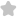Rearrange the following ( I to IV) ...
Question# Rearrange the following ( I to IV) in the order of increasing masses. (1) 0.5 mole of Og ((II) 6.5 gm molecûle of Nitrogen (III) 3.011 1023 molecule of O, (IV) 11.35 L of Co, at STP 02- (A) IV < III < II JEE/Engineering Exams Chemistry SolutionShare444.0 (1 ratings)5) ( 0.0 cdot 5 ) mole ( f cdot 0.3 equiv 0.5 times(16 times 3)(2 times 0.0) ) [ begin{array}{l} =frac{1}{2} times 48 text { potet } g =24 mathrm{g} end{array} ] (2) 0.5 gean motecule ( r ). ( n ) itrogen [ 11 / 1 ] equivalent 3 ( =frac{1}{2} x(16 times 2) g ) ( =169 ) ( therefore 0, operatorname{tin} n cdot(B) ) ( =frac{1}{2} times 44 g=229 )444.0 (1 ratings) Rate SolutionShareQuick and Stepwise Solutions Just click and SendOVER 20 LAKH QUESTIONS ANSWERED Download App for Free